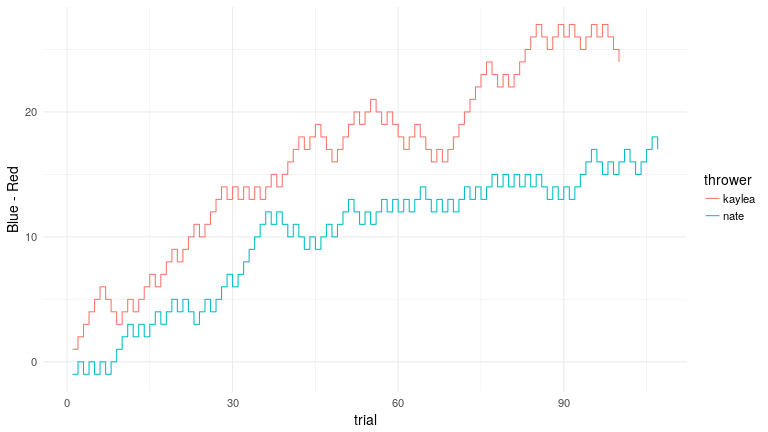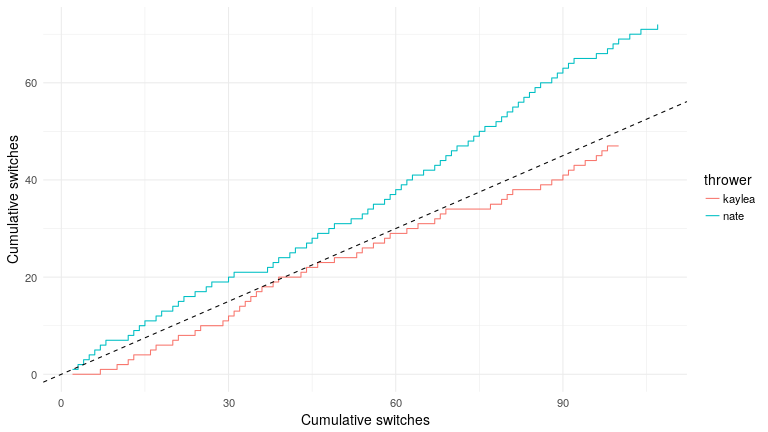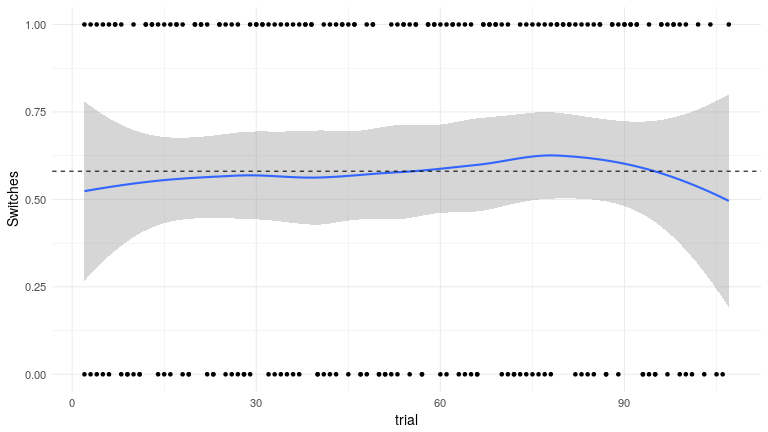# Statistical analysis of a switch pitch

This post analyzes a switch pitch toy as an exersize in data analysis. We will begin by using exact tests and some plots to understand whether the toy is a biased coin. Is it more likely to land in one of the two states? Exact tests are a very useful tool for working with categorical data because their assumptions are usually satisfied. We will also see how the switch pitch actually violates the assumptions of the exact tests.However, we will see that exchangability, the key assumption underlying these (any most other commonly used statistics) can be violated in the case of the switch pitch. Exchangeabiltiy says that the probability of the data does not depenend on the order of the data points. This is closely related to the commonly used ‘independent and identically distributed’ (iid) assumption.

A switch pitch is a neat toy that I found on Emilia’s desk in the community data lab at UW. It is a ball that when you toss it in the air sometimes, but not always changes color. It is a bit hard to describe so here is a short video I found on YouTube:

The person in the video manages to make the ball switch every time it is tossed. But when I was playing with the pitch switch, I found that I could not make it switch consistently. The mechanism by which the ball switchs was unclear. I began wondering if I had any ability to make the ball switch at all. Could it be a random process? Could my mind just be tricking my into thinking that I had power over the ball when in reality it was a coin switch?

I realized that I could answer these questions as a fun exercise in basic statistical analysis. The state of the ball is like a bernoulli random variable, but there might be dependence between one toss and the next. I recorded the state of the switch pitch for 107 tosses. While I started analyzing these 107 tosses, kaylea recorded 100 tosses of her own.

```  library(knitr)
library(data.table,quiet=T)
library(ggplot2)
opts_chunk\$set(fig.path = "images/figures/", dev='png',fig.width=16/1.5,fig.height=9/1.5)
```

I use the data.table package, which extends R data.frame. This will come in handy building variables. I also use ggplot2 for making plots.

```## my data was in ball_data.tsv
d\$blue <- d\$V1 == 'b'
d\$V1 <- NULL
d\$thrower <- 'nate'

kd\$blue <- kd\$V1=='b'
kd\$V1 <- NULL
kd\$thrower <- 'kaylea'
d <- rbindlist(list(d,kd),use.names=T)
```
```kd\$prev.blue <- shift(kd\$blue)
kd\$switches <- kd\$blue != kd\$prev.blue
d\$trial <- 1:nrow(d)
kd\$trial <- 1:nrow(kd)
kd\$blue.over.red <- cumsum(1*kd\$blue - 1*!kd\$blue)
```

## Is the ball biased? Does it prefer blue or red?

### Plot the cumulative advantage of blue over red

```d[,":="(blue.over.red = cumsum( (blue==TRUE) - (blue==FALSE) ),
trial = 1:.N
),
by='thrower']

ggplot(d,aes(y=blue.over.red,x=trial,group=thrower,color=thrower)) + geom_step() + scale_y_continuous("Blue - Red") + theme_minimal(base_size=14)
```From this plot we can see two things. First, the ball is blue more often than it is red for both Nate and Kaylea. Second, it seems like it is blue more often for Kaylea.

### Use binomial tests to evaluate uncertainty of these conclusions.

The binomial test, is an exact test that uses the cumulative density function (CDF) of the binomial distribution to give the probability of the data under a null hypothesis of the true probability of each event. It is a good test because it only has to assumes exchangeability, which means that each toss of the ball is independent from the others. We will reconsider this assumption in a minute.

```binom.test(sum(d\$blue),nrow(d))
```
```##
##  Exact binomial test
##
## data:  sum(d\$blue) and nrow(d)
## number of successes = 124, number of trials = 207, p-value =
## 0.005306
## alternative hypothesis: true probability of success is not equal to 0.5
## 95 percent confidence interval:
##  0.5288287 0.6663597
## sample estimates:
## probability of success
##              0.5990338
```

The ball is blue more often than it is red. And the binomial test tells us that this is probably not a result of random chance.

We can also use a statistical test to find out if Nate’s chances of getting a blue are statistically greater than Kaylea’s. In this case, Fisher’s exact test is approrpiate. Fisher’s exact test test the null hypothesis that the the two categories (Nate and Kaylea) have the same odds of obtaining a result (a blue or red toss) under the hypergeometric distribution. Like the binomial test, Fisher’s test is an exact test. This means that it only assumes that the data points are exchangeable. It doesn’t make any assumptions about how the data are distributed. To use Fisher’s test, we first create a contingency table.

```tab <- table(d[,.(blue,thrower)])
print(tab)
```
```##        thrower
## blue    kaylea nate
##   FALSE     38   45
##   TRUE      62   62
```

The table shows that while Kaylea and Nate caught the switch pitch when it was blue the same number of times. Nate caught it when it was red 7 more times than Kaylea. We pass the table into the `fisher.test` function to run the test.

```fisher.test(tab)
```
```##
##  Fisher's Exact Test for Count Data
##
## data:  tab
## p-value = 0.573
## alternative hypothesis: true odds ratio is not equal to 1
## 95 percent confidence interval:
##  0.4653861 1.5300592
## sample estimates:
## odds ratio
##  0.8451378
```

Since the Odds ratio in the data is well inside the 95% confidence interval, it seems that Kaylea was not statistically more likely to catch a blue than Nate was.

If we assume that the tosses are independent, then it seems clear that when thrown be either Kaylea or Nate, the switch pitch is more likely to land blue than to land red.

## Is the switch pitch more likely to switch than not?

However, it seems like the tosses may not be independent. If the switch pitch switches more or less than half the time, then the result of a given toss would depend on the state from which it was thrown. This would violate the exchangability assumption, which says that our observations are independent.

Let’s begin by looking at the data. The plot below shows the number of times the switch pitch has switched so far (the cumulative switches) on the y axis and the number of tosses so far (the cumulative tosses) on the x axis. The dashed line has a slope of 0.5 to show what the data would look like if the switch pitch switches half the time.

```d <- d[,prev.blue := shift(blue),by='thrower']
d <- d[,switches := prev.blue != blue]
d <- d[,cumulative.switches := c(NA,cumsum(switches[!is.na(switches)])),by='thrower']

p <- ggplot(d[!is.na(cumulative.switches)],aes(y=cumulative.switches,x=trial,group=thrower,color=thrower)) + geom_step() + scale_y_continuous("Cumulative switches") + scale_x_continuous("Cumulative switches")
p <- p + geom_abline(slope=0.5,intercept=0,linetype='dashed') + theme_minimal(base_size=14)
p
```When Nate tosses the ball, the odds appear to favor a switch. When Kaylea tosses the ball the odds look to be slightly against a switch. We can use our exact tests again to find out how much uncertainty we should have in these conclusions.

### Is the switch pitch more likely to switch than to not switch?

```binom.test(d\$switches)
```
```## Error in binom.test(d\$switches): 'x' must be nonnegative and integer
```

I observed the ball switch 68% of the time. The binomial test indicates that the observation that the ball was more likely to switch than to not switch is very probably not due to random chance. Since the ball is more likely to switch than to not switch, we can say that the state of the ball (red or blue) after one toss depends on the outcome of the previous toss.

## Is it the switch pitch, or just me?

It seems like whether the switch pitch switches or not depends on how it is tossed. Perhaps the probability of switching we have observed was because of the way that I was throwing it. Kaylea joined in the fun and recorded 100 of her own switch pitch tosses. Let’s see how things change when we add Kaylea’s data.

### Plotting the cumulative advantage of blue over red (again, this time with Kaylea’s data)

```ggplot(d,aes(y=blue.over.red,x=trial,group=thrower,color=thrower)) + geom_step() + scale_y_continuous("Blue - Red") + theme_minimal(base_size=14)
```Kaylea seems to get more blues, and more streaks than Nate.

### Is the ball still apparently unbiased?

```mean(d\$blue)
```
```##  0.5990338
```
```binom.test(sum(d\$blue),nrow(d))
```
```##
##  Exact binomial test
##
## data:  sum(d\$blue) and nrow(d)
## number of successes = 124, number of trials = 207, p-value =
## 0.005306
## alternative hypothesis: true probability of success is not equal to 0.5
## 95 percent confidence interval:
##  0.5288287 0.6663597
## sample estimates:
## probability of success
##              0.5990338
```

No! When we include Kaylea’s data, the binomial test indicates that our observation that the ball is more likely to be blue than to be red is probably not due to random chance. This seems pretty suprising! How could this be? Is the ball really biased?

### Is the ball still more likely to switch than not?

```binom.test(sum(d\$switches,na.rm=T),alternative='greater',nrow(d)-2)
```
```##
##  Exact binomial test
##
## data:  sum(d\$switches, na.rm = T) and nrow(d) - 2
## number of successes = 119, number of trials = 205, p-value =
## 0.01259
## alternative hypothesis: true probability of success is greater than 0.5
## 95 percent confidence interval:
##  0.5207551 1.0000000
## sample estimates:
## probability of success
##              0.5804878
```

No! With Kaylea’s data in the mix, we find that the probability of the ball switching falls closer to 0.5, and the difference from 0.5 might be due to random chance.

```binom.test(sum(d[thrower=='kaylea']\$switches,na.rm=T),alternative='greater',nrow(d[thrower=='kaylea'])-1)
```
```##
##  Exact binomial test
##
## data:  sum(d[thrower == "kaylea"]\$switches, na.rm = T) and nrow(d[thrower == "kaylea"]) - 1
## number of successes = 47, number of trials = 99, p-value = 0.7266
## alternative hypothesis: true probability of success is greater than 0.5
## 95 percent confidence interval:
##  0.3886011 1.0000000
## sample estimates:
## probability of success
##              0.4747475
```

Indeed, when Kaylea tosses the ball the odds of switching are pretty much even.

Since the number switches seems to grow linearly, it doesn’t seem like the probability of switching is increasing. We can check in another way.

```p <- ggplot(d[!is.na(switches)],aes(y=as.numeric(switches),x=trial)) + geom_point() + geom_smooth(method='loess') + scale_y_continuous("Switches")
p <- p + geom_abline(slope=0,intercept=mean(d\$switches,na.rm=T),linetype='dashed') + theme_minimal(base_size=14)
p
``````mod <- glm(switches ~ trial,data=d,family=binomial)
summary(mod)
```
```##
## Call:
## glm(formula = switches ~ trial, family = binomial, data = d)
##
## Deviance Residuals:
##    Min      1Q  Median      3Q     Max
## -1.369  -1.307   1.011   1.049   1.087
##
## Coefficients:
##             Estimate Std. Error z value Pr(>|z|)
## (Intercept) 0.211463   0.287776   0.735    0.462
## trial       0.002152   0.004772   0.451    0.652
##
## (Dispersion parameter for binomial family taken to be 1)
##
##     Null deviance: 278.85  on 204  degrees of freedom
## Residual deviance: 278.65  on 203  degrees of freedom
##   (2 observations deleted due to missingness)
## AIC: 282.65
##
## Number of Fisher Scoring iterations: 4
```